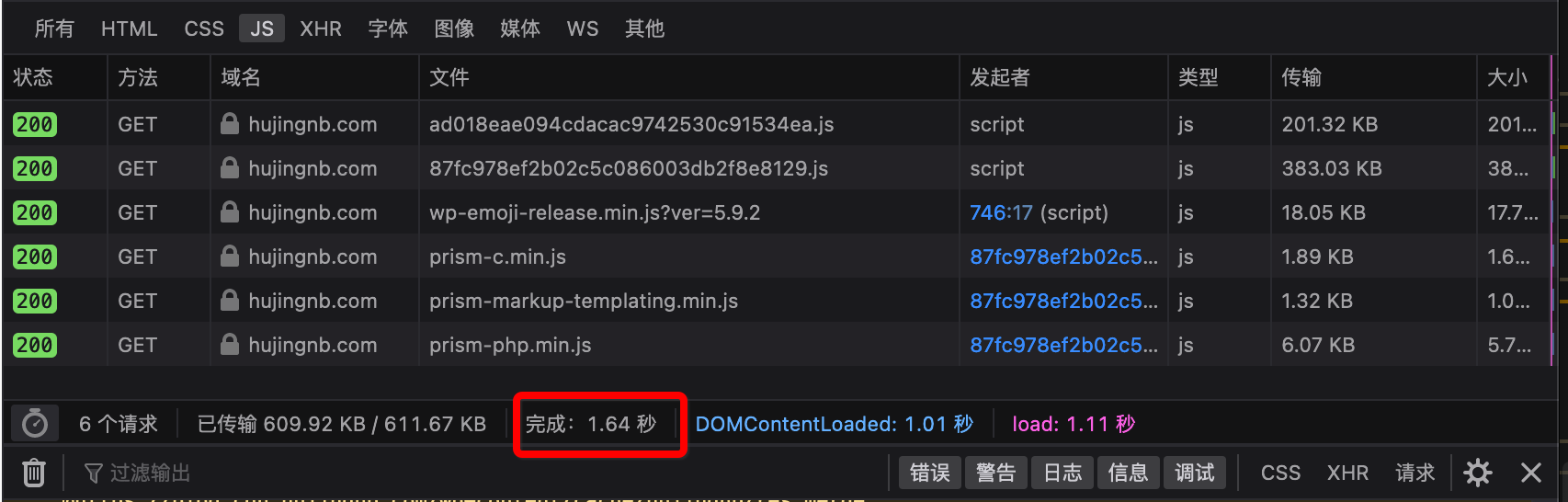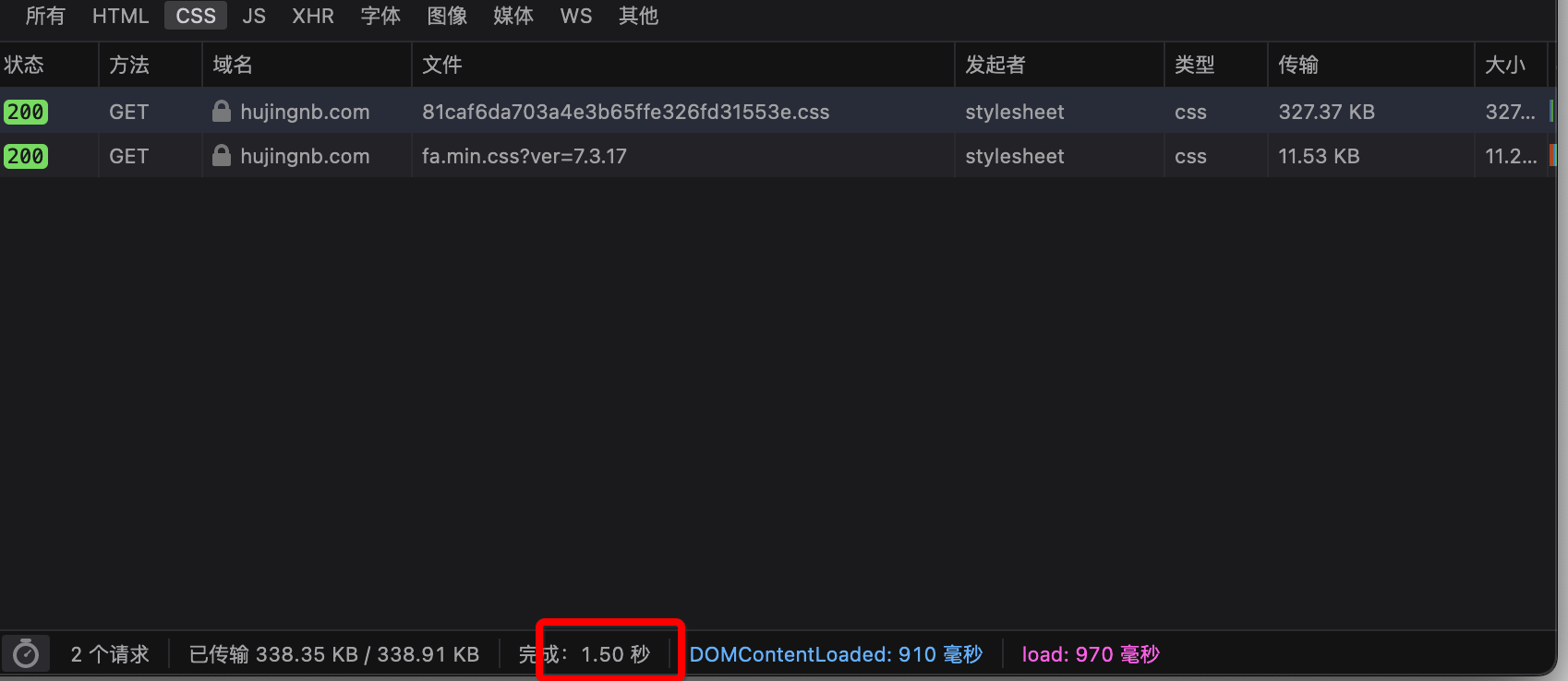# 前言# 合并 js 文件

<?php
// 开启页面缓冲
ob_start(function($content){ // 根据正则表达式, 将特定部分的 js 内容进行提取$mergePatternJsData = function ($content,$pattern, $replaceStr){ // 没有找到匹配的内容$noMatch = fn() =>  str_replace($replaceStr, "",$content);
// 将内容进行匹配提取
preg_match($pattern,$content, $matches); if(empty($matches)) return $noMatch(); // 匹配其中的 js 文件$pattern = "/<script\s*src='(.*)'.*><\/script>/U";
preg_match_all($pattern,$matches, $itemMatch); // 这里对页面中的部分 js 文件进行过滤 // 因为一些 js 文件中使用相对位置引入了其他资源文件 // 因此若是将其链接挪走了, 会导致引入资源文件失败$itemMatch = fileCantMergeFile($itemMatch); if(empty($itemMatch)) return $noMatch(); // 将匹配到的 js 文件进行合并, 并返回合并后的链接$replaceUrl = self::mergeResData($itemMatch); // 将原本的 js 内容去掉$content = str_replace($itemMatch, '',$content);
//  替换新的 js 文件
return str_replace($replaceStr, "<script src='{$replaceUrl}'></script>", $content); }; // JS_REPLACE_HEAD_STR/JS_REPLACE_FOOTER_STR 是提前在 header/footer 中放好的等待替换的字符串. 这里简单处理了, 其他处理方式也是可以的 // 这里说一下为什么 head 和 body 中的 js 资源不能进行合并 // 因为资源文件的加载时机不同, 因此不能进行合并 // 匹配 header 中的 js 文件$headerPattern = '/<head>[\S\s]*<\/head>/U';
$content =$mergePatternJsData($content,$headerPattern, self::JS_REPLACE_HEAD_STR);
// 匹配 body 中的 js
$bodyPattern = '/<body[\s>][\S\s]*<\/body>/U'; return$mergePatternJsData($content,$bodyPattern, self::JS_REPLACE_FOOTER_STR);
});

function mergeResData($urlList){ if(empty($urlList)) return null;
$workPath = __DIR__; // 项目路径, 自行修改 // 保存缓存文件的路径, 相对于项目的相对路径 // 这一, 这个路径要 nginx 可访问哦$relativePath = '';
// 获取合并后的本地缓存 js 文件
$filename = md5(implode('',$urlList)).'.js';
$filepath = "{$workPath}/{$relativePath}/{$filename}";
// 若缓存文件已经存在直接返回, 这里没有考虑 js 文件更新的情况. 若需要的话, 请自行处理
if(!file_exists($filepath)){ // 创建文件 // 提取所有文件的内容合集$allContent = '';
foreach ($urlList as$itemUrl){
$allContent .= PHP_EOL.file_get_contents($workPath.parse_url($itemUrl, PHP_URL_PATH)); }$dirname = dirname($filepath); if(!is_dir($dirname)) mkdir($dirname, 0777, true); file_put_contents($filepath, $allContent); } return "http://xxx.com/{$relativePath}/{$filename}"; } 如此一来, 就可以将页面中的 js 文件数量降低为2个了. 看一下效果, 效果还是十分显著的, js 数量减少了12个(多出来的是由 js 文件引入的 js), 时间减少了差不多0.9s# 合并 css 既然 js 文件可以合并, 那么自然, css 也能够合并. 合并的代码拿上面的改改就出来了. 这里直接上效果 在合并之前, css 文件9个. 合并后为2个(因为其中一个使用了相对路径), 时间减少了差不多0.1s 左右. 这里是因为 css 文件数量不多, 且文件本身较小, 所以效果没有 js 那么明显.js 和 css 都进行了合并, 难道就完了么? 不. 我们还可以对其进行压缩. # 压缩 js/css 找到了这个库: mrclay/minify . 直接composer require mrclay/minify 引进来就行. 使用如下方法在将内容写入到缓存文件时, 对其进行压缩: <?php // 压缩 js 内容$allContent = JSMin::minify($allContent) // 压缩 css 内容$allContent = Minify_CSSmin::minify(\$allContent)# 最终0 评论

0